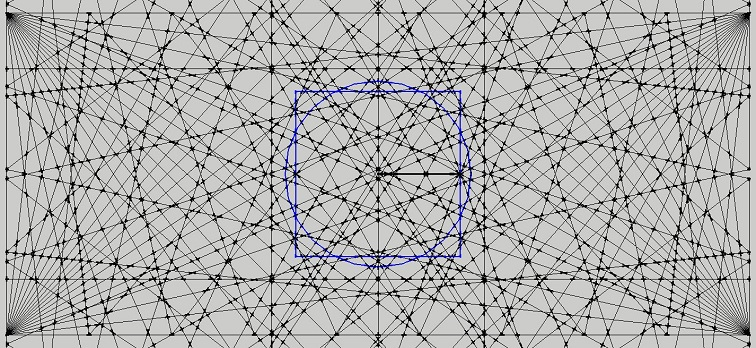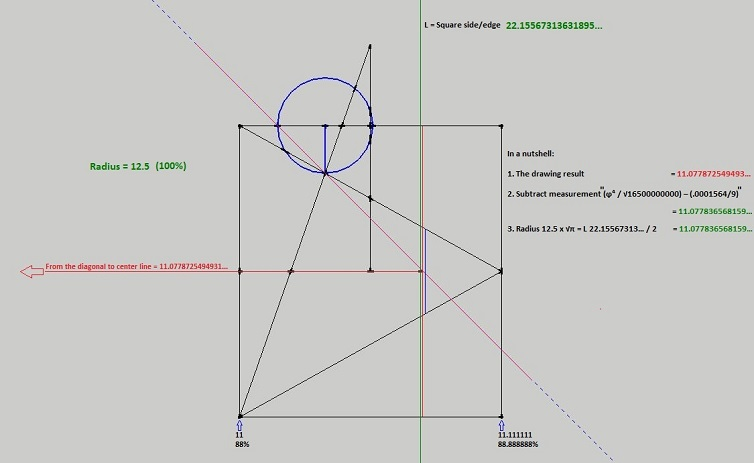Home | Study Links | Poems |

## The Silver Lining

This page was the result of me trying to calculate the central hinge point in this scissor-like shape.Ratios as measurement or percentage:
64.28… / 35.71…= 1.8
97.40… / 75.76…= 1.28571428…
75.76… - 21.64… / 21.64…= 2.5
75.76… / 21.64…= 3.5
97.40… / 21.64…= 4.5

The two hinge point numbers 3.142857... and its inverse 3.141602... are explained more in perfect ratios.Set side by side and facing straight on. Left a 2-d square and on the right a cube.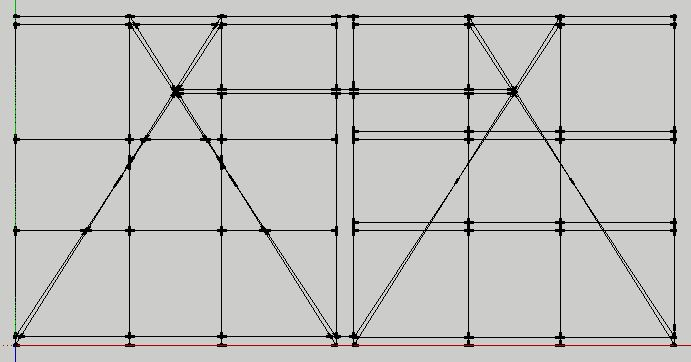By tilting them 1.485° forward, the back of the cube lifts up matching the 2-d drawing.
360 : 1.485 = 242.4242...(8000 : 33)A simple construction including the blue infinity line.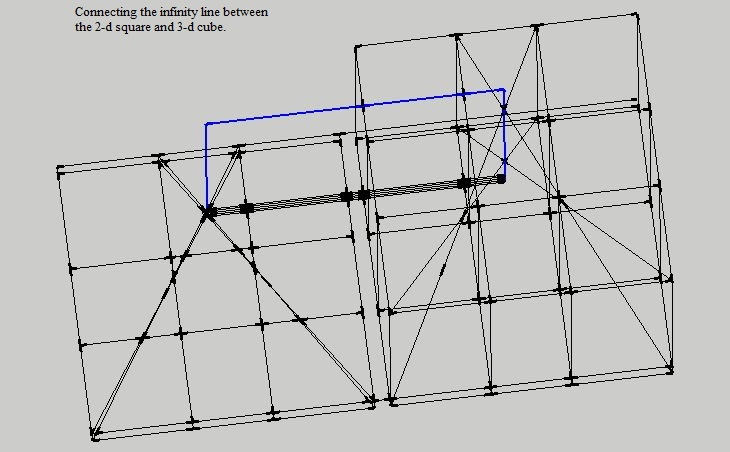It occurred to me that perhaps our physical reality is like a projection unto a screen as represented by the 2-d drawing. A mere reflection of the true reality which cannot be reached physically as represented by the 3-d or cube drawing. So, no matter where we are physically whether in space or earths’ surface, we always exist on a 2-d plane. Perhaps this is where the flat versus round earth idea originated.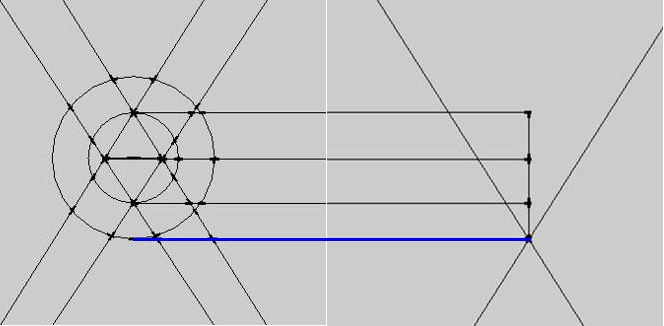Left and right side enlarged in a straight on view.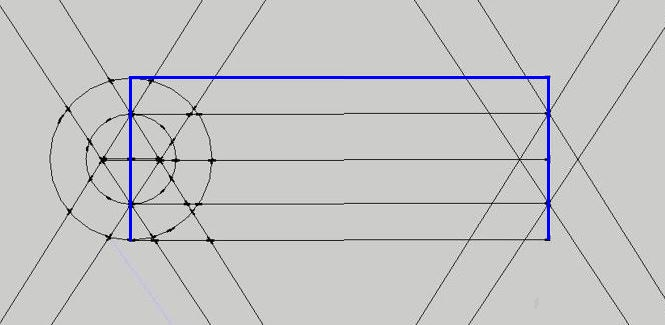Left and right side tilted, exactly matching the π⁴ line, suggesting a precision surveying or calibration devise.
Although interesting there is no way to calculate the central hinge point exactly and I changed tactic.Self explanatory as to how I did this and included are the pyramid lines. In this square all of the measurements between either vertical or horizontal grid points can be reduced to numbers without decimal point.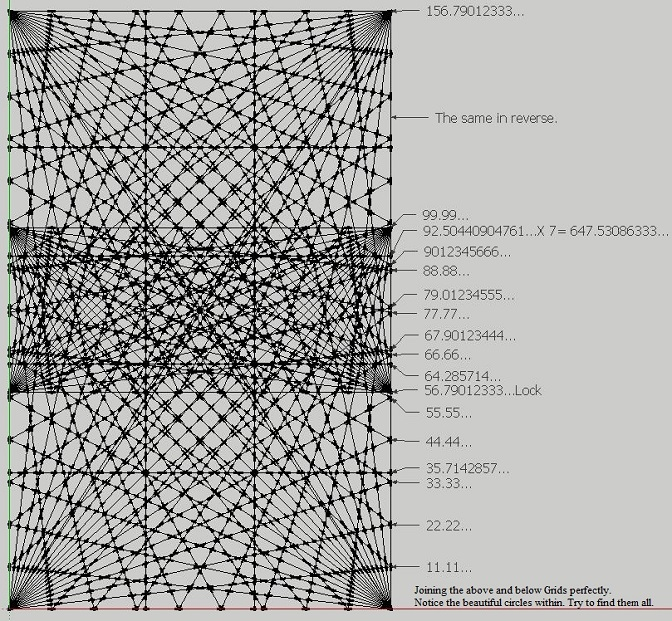At some point I realized that in order to move forward it requires opposite squares. Everything has an opposite.  This is where things get interesting, because how to know exactly where the two grids/nets join? Unless they are joined exactly the measurements between upper and lower grid points will not reduce to numbers without decimal points. However, match the upper and lower grids perfectly, and the numbers can be reduced to simple numbers not requiring decimal points. I have found a beautiful method not included here to do it. Also, if you look closely you can see a number of circles within the joined grid squares.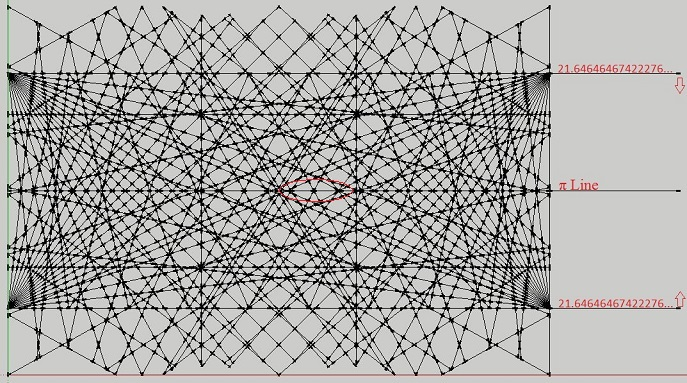From here on remember the number 21.64... (from diagram one). Circled in red and right side of the center line is the area of focus. Left of center line is its inverse and has the same information.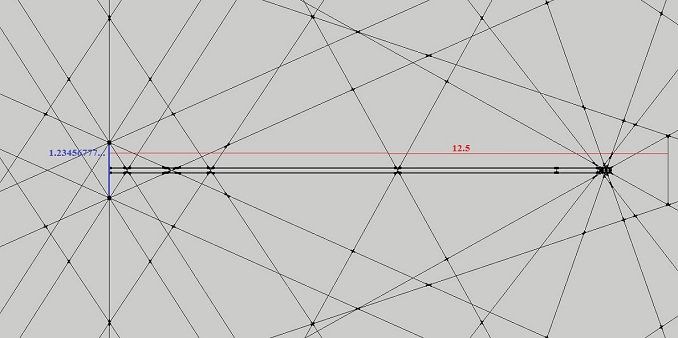Starting to zoom in to show beautiful measurements that can all be reduced to numbers without decimal places by multiplying mostly by 9 as well as a few other key numbers such as 63.Enlarging a bit more to show additional measurements.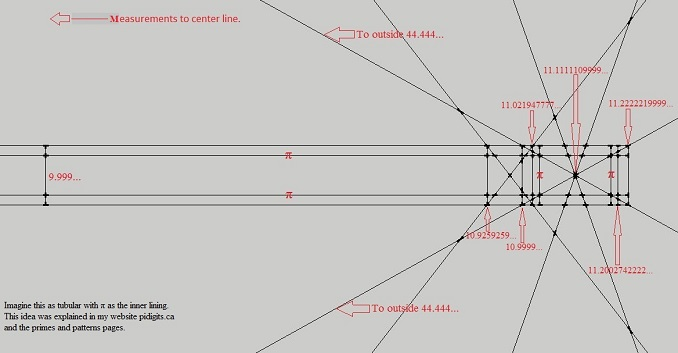Enlarging more and moving to the right. These measurements are to the center line. Here the idea of tube-like is apparent and where π represents the inner lining of a tube. Imagine looking at a opaque tube which is cut perfectly in half, straight-on from the side. The inside is not visible but angle it slightly and the inside lining is now visible. Naturally, all measurements in these drawings are known except the π lines, which were added because we know what π is and can be used to reverse engineer this design.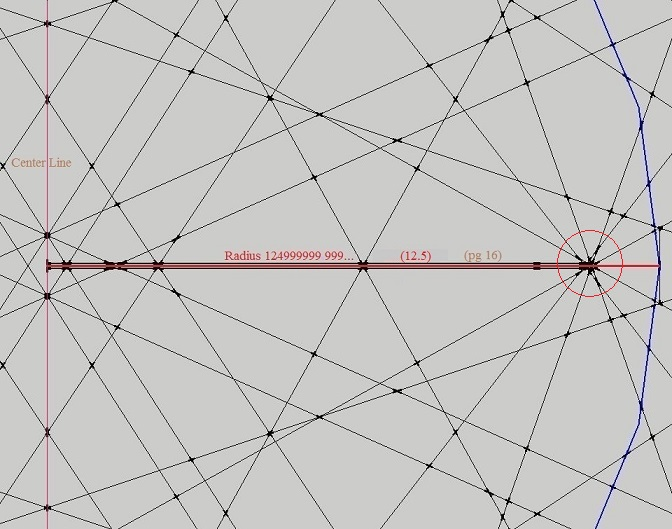Not seeking this, but at this point I realized that I am dealing with equalizing the square and circle area as well.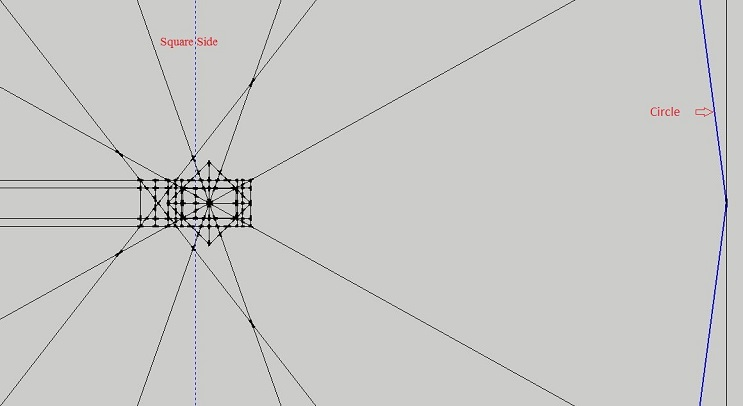The red circle enlarged.Enlarged further and looking like the eye of a needle.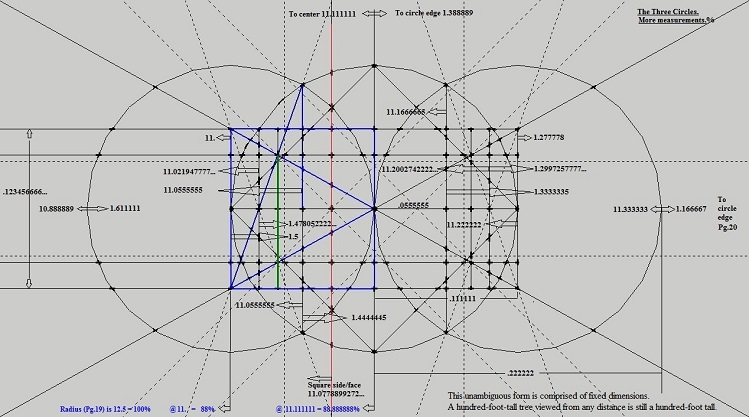The three circles and measurements to the left center line and right main circle edge (several diagrams up).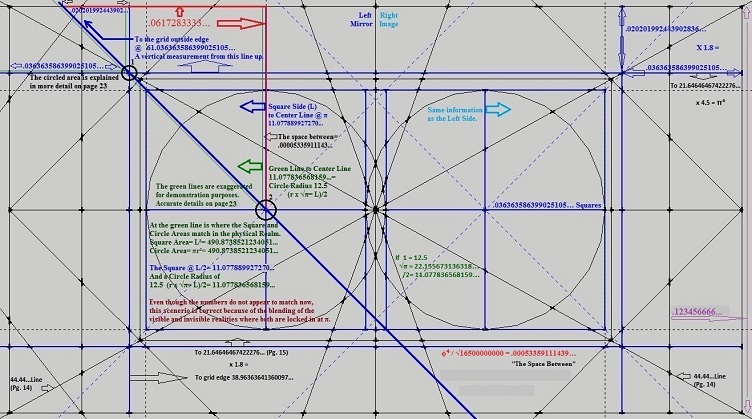More detail.Black circled areas 1 and 2 from previous diagram enlarged. And the The space between explanation.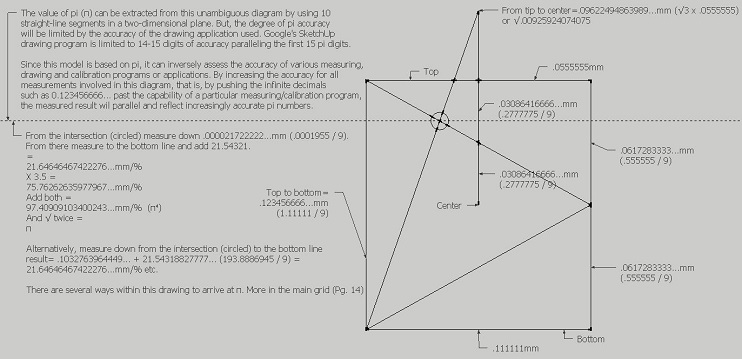See the three circles diagram.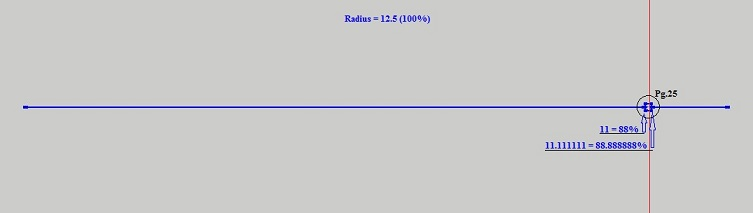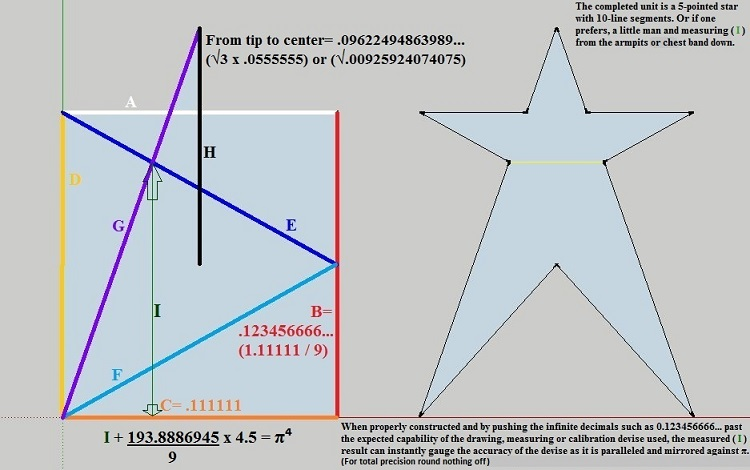This equation and geometric construction to π is simple and easy to reproduce. Whether hand drawn on paper, using Google Sketchup or some other drawing program, or laid out on a level field as a surveying exercise, this design will reflect its exactness by the number of correct π decimal places extracted from it.
A fun challenge for anyone to see how many π decimal places they can attain. I foresee this diagram to be used as an educational, hands-on and learning about pi project for children.
Google SketchUp has a limit of 15-16 or so accurate measurement digits, and it parallels the amount of pi digits. Even with a hand drawn diagram using only a pencil and straightedge one can construct π to an accuracy of about 5 decimal places. The decimal places can be moved depending on the size of the diagram, but obviously all of the numbers that belong to the diagram must have their decimal places moved by the same amount. Once the " I " measurement has been established the decimal must be moved in front of it to complete the equation.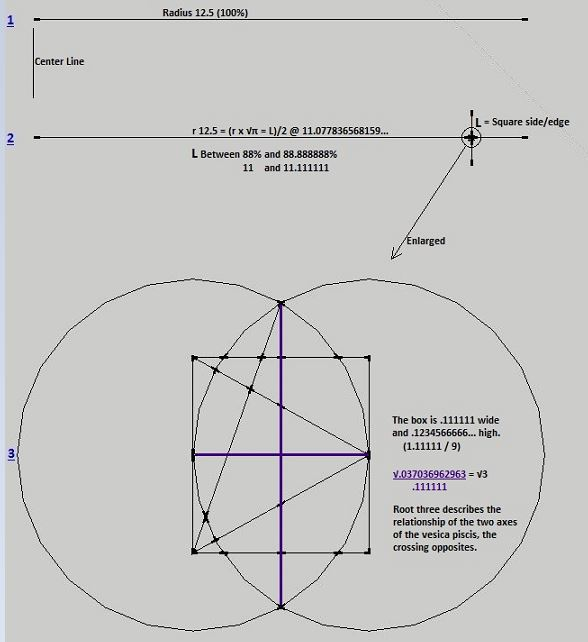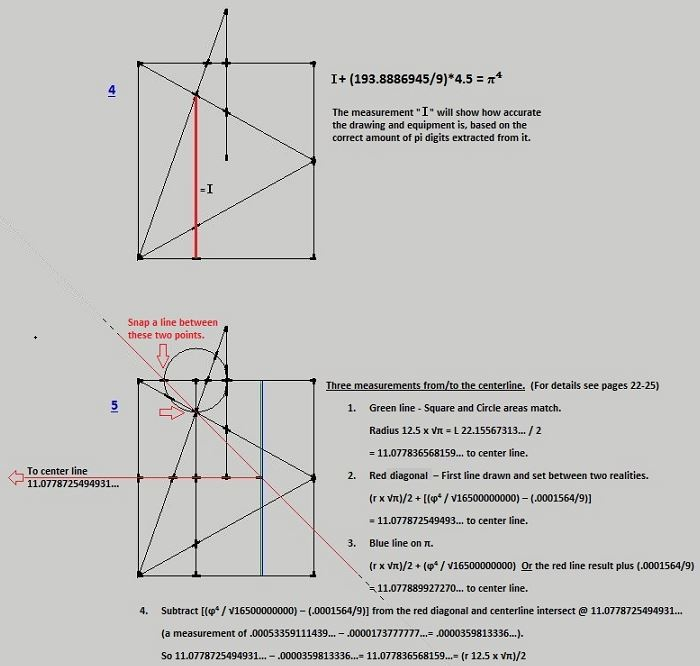This method based on the constant π can be used as the standard to which the accuracies of the various measuring, drawing and calibration programs can be compared. In other words, measurements can be instantly compared to pi digits because they parallel them. At the decimal place where pi digits go wrong the measurement is also inaccurate. For example, the distance of a moving target is instantly gauged against π for accuracy.